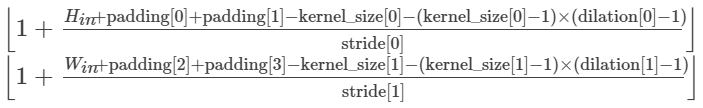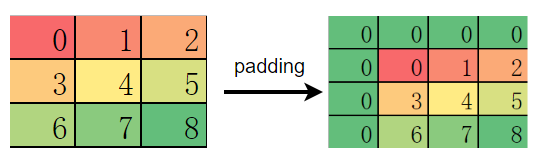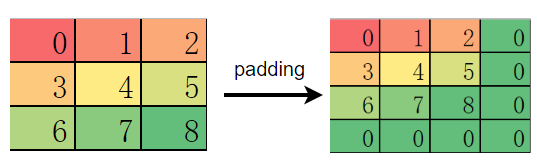# 与PyTorch典型区别¶

## 与PyTorch典型接口区别¶

### torch.device¶

PyTorch 在构建模型时，通常会利用 torch.device 指定模型和数据绑定的设备，是在 CPU 还是 GPU 上，如果支持多 GPU，还可以指定具体的 GPU 序号。绑定相应的设备后，需要将模型和数据部署到对应设备，代码如下：

import os
import torch
from torch import nn

# bind to the GPU 0 if GPU is available, otherwise bind to CPU
device = torch.device("cuda:0" if torch.cuda.is_available() else "cpu") # 单 GPU 或者 CPU
# deploy model to specified hardware
model.to(device)
# deploy data to specified hardware
data.to(device)

# distribute training on multiple GPUs
if torch.cuda.device_count() > 1:
model = nn.DataParallel(model, device_ids=[0,1,2])
model.to(device)

# set available device
os.environ['CUDA_VISIBLE_DEVICE']='1'
model.cuda()


import mindspore as ms
ms.set_context(device_target='Ascend', device_id=0)

# define net
Model = ..
# define dataset
dataset = ..
# training, automatically deploy to Ascend according to device_target
Model.train(1, dataset)


### nn.Module¶

MindSpore 中的 nn.Cell 类发挥着和 PyTorch 中 nn.Module 相同的作用，都是用来构建图结构的模块，MindSpore 也同样提供了丰富的 API 供开发者使用，虽然名字不能一一对应，但 nn.Module 中常用的功能都可以在nn.Cell 中找到映射。

nn.Module

nn.Cell

named_children

cells_and_names

insert_child_to_cell

parameters

get_parameters

### 数据对象¶

MindSpore 优化了数据对象的设计逻辑，仅保留了两种数据对象：TensorParameter，其中 Tensor 对象仅参与运算，并不需要对其进行梯度求导和参数更新，而 Parameter 数据对象和 PyTorch 的 Parameter 意义相同，会根据其属性requires_grad 来决定是否对其进行梯度求导和 参数更新。 在网络迁移时，只要是在 PyTorch 中未进行参数更新的数据对象，均可在 MindSpore 中声明为 Tensor

## 与PyTorch典型算子区别¶

MindSpore 的大部分算子 API 和 TensorFlow 相近，但也有一部分算子的默认行为与 PyTorch 或 TensorFlow 存在差异。开发者在做网络脚本迁移时，如果未注意到这些差异，直接使用默认行为，很容易出现与原迁移网络不一致的情况，影响网络训练，我们建议开发者在迁移网络时不仅对齐使用的算子，也要对齐算子的属性。这里我们总结了几个常见的差异算子。

### nn.Dropout¶

Dropout 常用于防止训练过拟合，有一个重要的 概率值 参数，该参数在 MindSpore 中的意义与 PyTorch 和 TensorFlow 中的意义完全相反。

### nn.BatchNorm2d¶

BatchNorm 是 CV 领域比较特殊的正则化方法，它在训练和推理的过程中有着不同计算流程，通常由算子属性控制。MindSpore 和 PyTorch 的 BatchNorm 在这一点上使用了两种不同的参数组。

• 差异一

torch.nn.BatchNorm2d 在不同参数下的状态

training

track_running_stats

True

True

True

False

False

True

False

False

mindspore.nn.BatchNorm2d 在不同参数下的状态

use_batch_statistics

True

Fasle

None

• 差异二

BatchNorm系列算子 的 momentum 参数在 MindSpore 和 PyTorch 表示的意义相反，关系为：

$momentum_{pytorch} = 1 - momentum_{mindspore}$

### ops.Transpose¶

:

# PyTorch 代码
import numpy as np
import torch
from torch import Tensor
data = np.empty((1, 2, 3, 4)).astype(np.float32)
ret1 = torch.transpose(Tensor(data), dim0=0, dim1=3)
print(ret1.shape)
ret2 = Tensor(data).permute((3, 2, 1, 0))
print(ret2.shape)

torch.Size([4, 2, 3, 1])
torch.Size([4, 3, 2, 1])


MindSpore 和 TensorFlow 的 transpose算子功能相同， 虽然名字叫 transpose，但实际可以做多个轴的同时变换，等价于 Tensor.permute。因此，MindSpore 也不再单独提供类似 torch.tranpose 的算子。

:

# mindspore 代码
import mindspore as ms
import numpy as np
from mindspore import ops
ms.set_context(device_target="CPU")
data = np.empty((1, 2, 3, 4)).astype(np.float32)
ret = ops.Transpose()(ms.Tensor(data), (3, 2, 1, 0))
print(ret.shape)

(4, 3, 2, 1)


### Conv 和 Pooling¶:

# mindspore example
import numpy as np
import mindspore as ms
from mindspore import ops
ms.set_context(device_target="CPU")
data = np.arange(9).reshape(1, 1, 3, 3).astype(np.float32)
print(data)
print(op(ms.Tensor(data)))

[[[[0. 1. 2.]
[3. 4. 5.]
[6. 7. 8.]]]]
[[[[4. 5.]
[7. 8.]]]]


:

import numpy as np
import mindspore as ms
from mindspore import ops
ms.set_context(device_target="CPU")
data = np.arange(9).reshape(1, 1, 3, 3).astype(np.float32)
# only padding on top left of feature map
print(res)

[[[[0. 2.]
[6. 8.]]]]


### 默认权重初始化不同¶

• Conv2d

• mindspore.nn.Conv2d的weight为：$$\mathcal{N}(0, 1)$$，bias为：zeros。

• torch.nn.Conv2d的weight为：$$\mathcal{U} (-\sqrt{k},\sqrt{k} )$$，bias为：$$\mathcal{U} (-\sqrt{k},\sqrt{k} )$$

• tf.keras.Layers.Conv2D的weight为：glorot_uniform，bias为：zeros。

其中，$$k=\frac{groups}{c_{in}*\prod_{i}^{}{kernel\_size[i]}}$$

• Dense(Linear)

• mindspore.nn.Linear的weight为：$$\mathcal{N}(0, 1)$$，bias为：zeros。

• torch.nn.Dense的weight为：$$\mathcal{U}(-\sqrt{k},\sqrt{k})$$，bias为：$$\mathcal{U}(-\sqrt{k},\sqrt{k} )$$

• tf.keras.Layers.Dense的weight为：glorot_uniform，bias为：zeros。

其中，$$k=\frac{groups}{in\_features}$$

## 与PyTorch执行流程区别¶

### 自动微分¶

MindSpore 和 PyTorch 都提供了自动微分功能，让我们在定义了正向网络后，可以通过简单的接口调用实现自动反向传播以及梯度更新。但需要注意的是，MindSpore 和 PyTorch 构建反向图的逻辑是不同的，这个差异也会带来 API 设计上的不同。

#### PyTorch 的自动微分¶

:

import torch
x = x * 2
y = x - 1
y.backward(x)


#### MindSpore的自动微分¶

MindSpore 在做自动微分时，需要传入正向图结构，自动微分的过程就是通过对正向图的分析从而得到反向传播信息，自动微分的结果与正向计算中具体的数值无关，仅和正向图结构有关。通过对正向图的自动微分，我们得到了反向传播过程，而这个反向传播过程其实也是通过一个图结构来表达，也就是反向图。将反向图添加到用户定义的正向图之后，组成一个最终的计算图。不过后添加的反向图和其中的反向算子我们并不感知，也无法手动添加，只能通过 MindSpore 为我们提供的接口自动添加，这样做也避免了我们在反向构图时引入错误。

:

import mindspore as ms
from mindspore import nn, ops

def __init__(self, net):
self.net = net

def construct(self, x, y):


### 学习率更新¶

#### PyTorch的学习率更新策略¶

PyTorch提供了torch.optim.lr_scheduler包用于动态修改lr，使用的时候需要显式地调用optimizer.step()scheduler.step()来更新lr，详情请参考如何调整学习率

#### MindSpore的学习率更新策略¶

MindSpore的学习率是包到优化器里面的，每调用一次优化器，学习率更新的step会自动更新一次，详情请参考学习率与优化器

### 分布式场景¶

#### PyTorch的分布式设置¶

import os
import torch
import torch.distributed as dist
import torch.multiprocessing as mp
import torch.nn as nn
import torch.optim as optim
from torch.nn.parallel import DistributedDataParallel as DDP

def example(rank, world_size):
# create default process group
dist.init_process_group("gloo", rank=rank, world_size=world_size)
# create local model
model = nn.Linear(10, 10).to(rank)
# construct DDP model
ddp_model = DDP(model, device_ids=[rank])
# define loss function and optimizer
loss_fn = nn.MSELoss()
optimizer = optim.SGD(ddp_model.parameters(), lr=0.001)

# forward pass
outputs = ddp_model(torch.randn(20, 10).to(rank))
labels = torch.randn(20, 10).to(rank)
# backward pass
loss_fn(outputs, labels).backward()
# update parameters
optimizer.step()

def main():
world_size = 2
mp.spawn(example,
args=(world_size,),
nprocs=world_size,
join=True)

if __name__=="__main__":
# Environment variables which need to be
# set when using c10d's default "env"
# initialization mode.
os.environ["MASTER_PORT"] = "29500"
main()


#### MindSpore的分布式设置¶

MindSpore的分布式配置是使用运行时配置项的，详情请参考分布式并行，如：

import mindspore as ms
from mindspore.communication.management import init, get_rank, get_group_size

# 初始化多卡环境
init()

# 获取组成分布式场景的卡数和当前进程的逻辑id
group_size = get_group_size()
rank_id = get_rank()

# 配置数据并行模式运行分布式场景